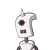# the perimeters of 2 similar triangles are 25 cm and 15 cm respectively if one side of the 1st triangle is 9 cm then the correspond

the perimeters of 2 similar triangles are 25 cm and 15 cm respectively if one side of the 1st triangle is 9 cm then the corresponding side of the 2nd triangle is

### 1 thought on “the perimeters of 2 similar triangles are 25 cm and 15 cm respectively if one side of the 1st triangle is 9 cm then the correspond”

1.Step-by-step explanation:

In similar triangles, the perimeter of the triangles will be in the ratio of their corresponding sides.

The ratio of their perimeter is

perimeterofΔ1. = 25

perimeterofΔ2.= 15

So, the side of triangle2 will be

9. = 25

sideofΔ2. = 15

⟹sideofΔ2=

9∗15

25

=5.4.